Question 14. Solve the following example. The masses of the earth and moon are 6 x 1024 kg and 7.4x1022 kg, respectively. The distance between them is 3.84 x 105 km. Calculate the gravitational force of attraction between the two?

Question 14.

Solve the following example.

The masses of the earth and moon are 6 x 1024 kg and 7.4x1022 kg, respectively. The distance between them is 3.84 x 105 km. Calculate the gravitational force of attraction between the two?

Use G = 6.7 x 10-11 N m2 kg-2

Gravitational force of attraction between two bodies of masses m1 and m2separated by a distance of R is given by;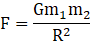G = Gravitational constant;

Figure below illustrates the question well;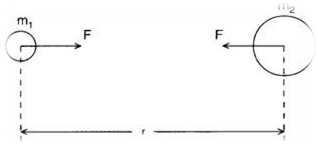Putting values of m1 =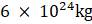;

m2 =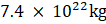;

R =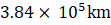=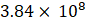m (since 1km = 1000m);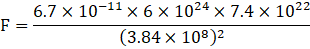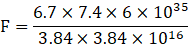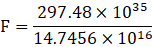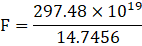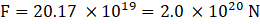Therefore the gravitational force of attraction between the earth and the moon is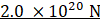.